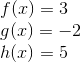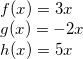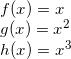### Day 14 - Derivative Rules - 01.27.15

Update
• New Seats! Sit in a different pod!
• Unit 2 Test on Friday, 2/13!

Bell Ringer

1. Find the derivative of the following:2. Find the derivative of the following:2. Find the derivative of the following:Review

Lesson
• Derivative Rules
• Constant Rule
• Power Rule
• Constant Multiple Rule
• Derivative of Sine and Cosine Functions
• Rates of Change
• Position Function
• Velocity Function
• Acceleration Function

Exit Ticket
• Posted on the board at the end of the block
Lesson Objectives
• How are tangent lines related to secant lines?
• How are derivatives related to tangent lines?
• How are derivative calculated?

#### In-Class Help Requests

Standard(s)
• APC.5
• Investigate derivatives presented in graphic, numerical, and analytic contexts and the relationship between continuity and differentiability.
• The derivative will be defined as the limit of the difference quotient and interpreted as an instantaneous rate of change.
• APC.6
• ​The student will investigate the derivative at a point on a curve.
• Includes:
• finding the slope of a curve at a point, including points at which the tangent is vertical and points at which there are no tangents
• using local linear approximation to find the slope of a tangent line to a curve at the point
• ​defining instantaneous rate of change as the limit of average rate of change
• approximating rate of change from graphs and tables of values.
• APC.7
• Analyze the derivative of a function as a function in itself.
• Includes:
• comparing corresponding characteristics of the graphs of f, f', and f''
• ​defining the relationship between the increasing and decreasing behavior of f and the sign of f'
• ​translating verbal descriptions into equations involving derivatives and vice versa
• defining the relationship between the concavity of f and the sign of f "
• APC.9
• Apply formulas to find derivatives.
• Includes:
• derivatives of algebraic and trigonometric functions
• derivations of sums, products, quotients, inverses, and composites (chain rule) of elementary functions
• derivatives of implicitly defined functions
• higher order derivatives of algebraic and trigonometric functions

Past Checkpoints